Next: Plasma Dispersion Function Up: Waves in Warm Plasmas Previous: Landau Damping

# Physics of Landau Damping

We have explained Landau damping in terms of mathematics. Let us now consider the physical explanation for this effect. The motion of a charged particle situated in a one-dimensional electric field varying as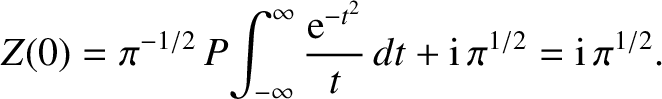is determined by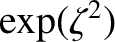(1016)

Since we are dealing with a linearized theory in which the perturbation due to the wave is small, it follows that if the particle starts with velocity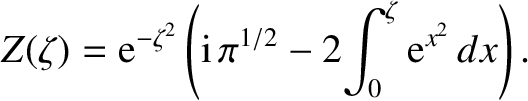at position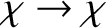then we may substitute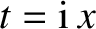for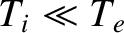in the electric field term. This is actually the position of the particle on its unperturbed trajectory, starting at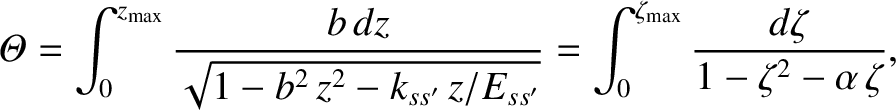at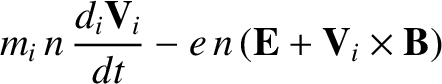. Thus, we obtain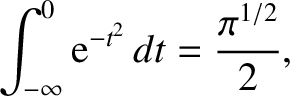(1017)

which yields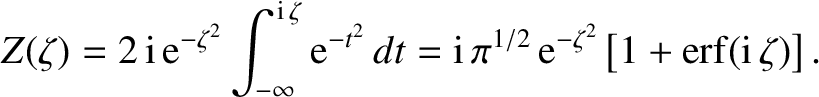(1018)

As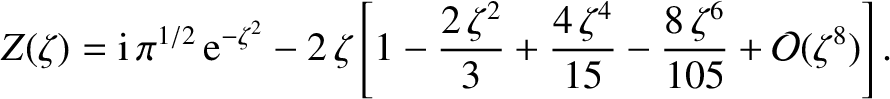, the above expression reduces to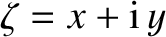(1019)

showing that particles withclose to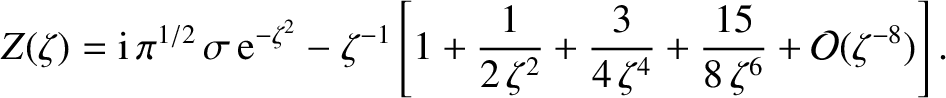, that is with velocity components along the-axis close to the phase velocity of the wave, have velocity perturbations which grow in time. These so-called resonant particles gain energy from, or lose energy to, the wave, and are responsible for the damping. This explains why the damping rate, given by Eq. (1014), depends on the slope of the distribution function calculated at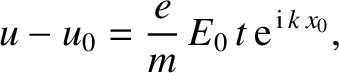. The remainder of the particles are non-resonant, and have an oscillatory response to the wave field.

To understand why energy should be transferred from the electric field to the resonant particles requires more detailed consideration. Whether the speed of a resonant particle increases or decreases depends on the phase of the wave at its initial position, and it is not the case that all particles moving slightly faster than the wave lose energy, whilst all particles moving slightly slower than the wave gain energy. Furthermore, the density perturbation is out of phase with the wave electric field, so there is no initial wave generated excess of particles gaining or losing energy. However, if we consider those particles which start off with velocities slightly above the phase velocity of the wave then if they gain energy they move away from the resonant velocity whilst if they lose energy they approach the resonant velocity. The result is that the particles which lose energy interact more effectively with the wave, and, on average, there is a transfer of energy from the particles to the electric field. Exactly the opposite is true for particles with initial velocities lying just below the phase velocity of the wave. In the case of a Maxwellian distribution there are more particles in the latter class than in the former, so there is a net transfer of energy from the electric field to the particles: i.e., the electric field is damped. In the limit as the wave amplitude tends to zero, it is clear that the gradient of the distribution function at the wave speed is what determines the damping rate.

It is of some interest to consider the limitations of the above result, in terms of the magnitude of the initial electric field above which it is seriously in error and nonlinear effects become important. The basic requirement for the validity of the linear result is that a resonant particle should maintain its position relative to the phase of the electric field over a sufficiently long time for the damping to take place. To obtain a condition that this be the case, let us consider the problem in the frame of reference in which the wave is at rest, and the potential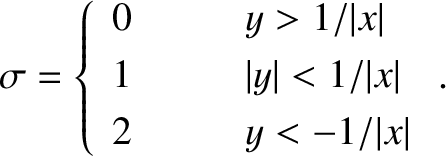seen by an electron is as sketched in Fig. 35.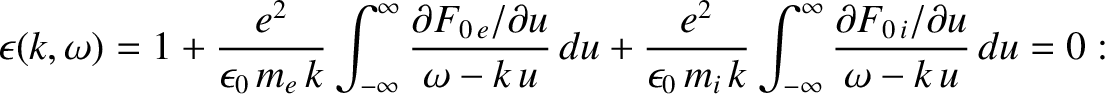If the electron starts at rest (i.e., in resonance with the wave) atthen it begins to move towards the potential minimum, as shown. The time for the electron to shift its position relative to the wave may be estimated as the period with which it bounces back and forth in the potential well. Near the bottom of the well the equation of motion of the electron is written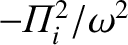(1020)

where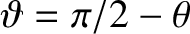is the wave-number, and so the bounce time is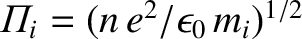(1021)

where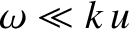is the amplitude of the electric field. We may expect the wave to damp according to linear theory if the bounce time,, given above, is much greater than the damping time. Since the former varies inversely with the square root of the electric field amplitude, whereas the latter is amplitude independent, this criterion gives us an estimate of the maximum allowable initial perturbation which is consistent with linear damping.

If the initial amplitude is large enough for the resonant electrons to bounce back and forth in the potential well a number of times before the wave is damped, then it can be demonstrated that the result to be expected is a non-monotonic decrease in the amplitude of the electric field, as shown in Fig. 36. The period of the amplitude oscillations is similar to the bounce time,.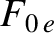Next: Plasma Dispersion Function Up: Waves in Warm Plasmas Previous: Landau Damping
Richard Fitzpatrick 2011-03-31